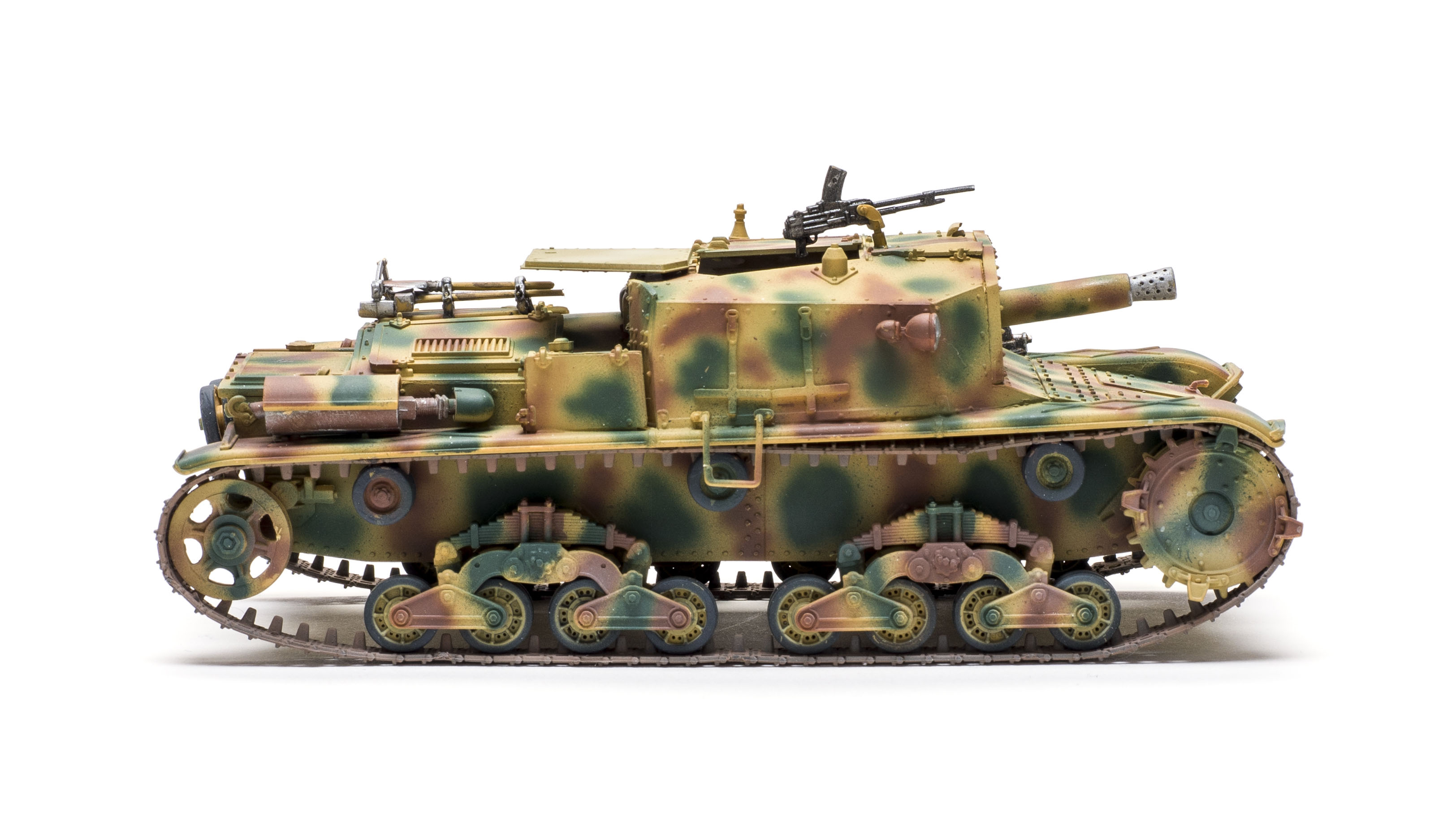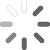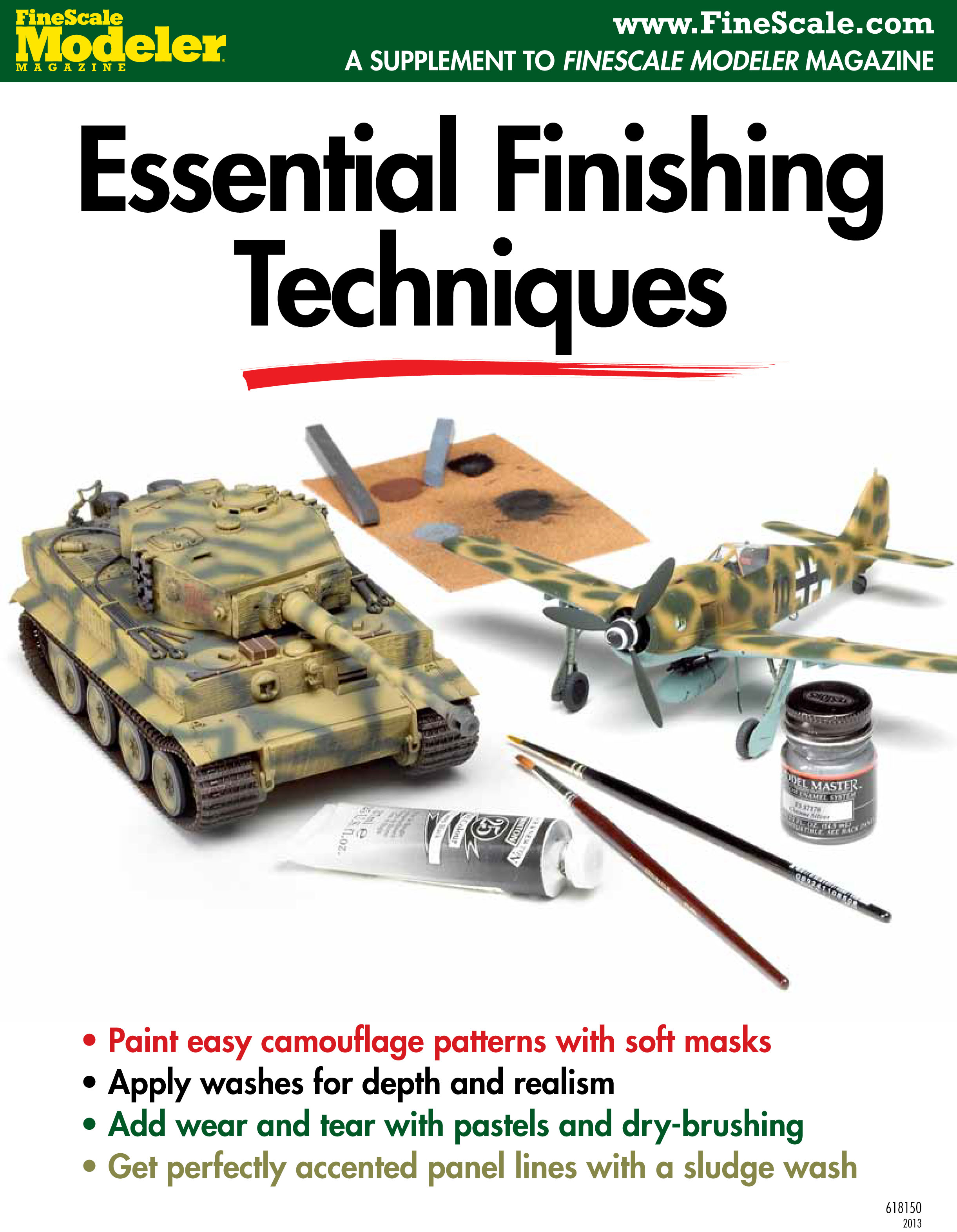## SEARCH FINESCALE.COM

Enter keywords or a search phrase below:

# Convert scale model ratios

It’s not as hard as it sounds
RELATED TOPICS: MODELING TOOLS
As modelers, we often find ourselves working with either scale drawings or photographs as references. Often, those drawings or photos don’t depict our subject in the same scale as our model. Sometimes, we’re able to enlarge or reduce an image on a computer, but what if you can’t do that? There is another way, but it involves a little bit of math—or at least plugging the numbers into your smartphone’s calculator and letting it do the work for you.

Let’s begin with the assumption that you have a scale drawing. The key to working with a scale drawing is the “scale multiplier factor,” which is a number that expresses the proportional difference between the drawing and your model. Once you have this factor, you simply enter any dimension from the drawing into your calculator, multiply by the factor, and out comes a corresponding dimension for your model.

First, determine the decimal equivalents for the scale of the drawing and the scale of your model: divide the numerator (top number in a fraction) by the denominator (bottom number in a fraction). For example, to get the decimal equivalent for 1/35 (1:35) scale, divide 1 by 35 and you get .0286 (rounded up). For easy reference, the Scale Conversion Chart shows decimal equivalents for common modeling scales.

To find the scale multiplier factor, divide the model’s decimal scale equivalent by that of your drawing’s. For example, if our model is 1/35 scale, we found our decimal equivalent to be .0286. Let’s say our scale drawing is in 1/48 scale. Its decimal equivalent is .0208. To find the scale multiplier factor, we divide .0286 (the model) by .0208 (the drawing) and get 1.375. All we have to do is multiply any measurement off the drawing by 1.375 to get the corresponding dimension for our 1/35 scale model.

As an easy example, if a dimension on the 1/48 scale drawing measures 1 inch long, then the same part we’re making for our 1/35 scale model must be 1.375 inches long.

Maybe you don’t have a scale drawing, but you do have a model that you are confident is accurate and know it’s scale. You use the same process.

What if we don’t know the scale of a drawing? Well, there’s a way around that. Convert a real dimension of the vehicle (its length, for instance) and convert from feet to inches (multiply by 12) or meters to centimeters and then divide by the same dimension measured on the drawing. This will give us the drawing’s scale, and we can find the scale multiplier as shown above.

For example, let’s assume our research on a boat we want to build is 40 feet long; 40 x 12 gives us 480 inches. We measure the length of the boat in the drawing and find it is 10 inches long, giving us a fraction of 10/480 or 1/48 scale. Proceed as we’ve already discussed.

Finally, let’s assume we have no information about the dimensions of the real vehicle nor the scale of the drawings we are working from, but are willing to assume the dimensions of our model are accurate. Take a dimension (say, the overall length) of the model and divide it by the same dimension from the drawing. This will give us the scale multiplier without the intermediate step. This process is particularly useful when scaling measurements from photographs. However, only direct side views should be used for this because any change in perspective will considerably distort the dimensions.

And there you have it: Everything you need to know about converting scales!

Excerpted from Shep Paine’s Armor Modelers Guide.

JOIN THE DISCUSSION

## SPECIAL ISSUES & MORE